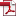• Journal of Internet Computing and Services
ISSN 2287 - 1136(Online) / ISSN 1598 - 0170 (Print)
http://jics.or.kr/

Optimization of Bi-criteria Scheduling using Genetic Algorithms

Journal of Internet Computing and Services, Vol. 6, No. 6, pp. 99-106, Dec. 2005
Full Text:Keywords: genetic algorithm, multiprocessor scheduling, simulated annealing

Abstract

The task scheduling in multiprocessor system Is one of the key elements in the effective utilization of multiprocessor systems. The optimal assignment of tasks to multiprocessor is, in almost all practical cases, an NP hard problem. Consequently various modern heuristics based algorithms have been proposed for practical reason. Recently, several approaches using Genetic Algorithm (GA) are proposed. However, these algorithms have only one objective such as minimizing cost and makespan. This paper proposes a new task scheduling algorithm using Genetic Algorithm combined simulated annealing (GA+SA) on multiprocessor environment. In solution algorithms, the Genetic Algorithm (GA) and the simulated annealing (SA) are cooperatively used. In this method. the convergence of GA is improved by introducing the probability of SA as the criterion for acceptance of new trial solution. The objective of proposed scheduling algorithm is to minimize makespan and total number of processors used. The effectiveness of the proposed algorithm is shown through simulation studies. In simulation studies, the results of proposed algorithm show better than that of other algorithms.

Statistics
Show / Hide Statistics

Statistics (Cumulative Counts from November 1st, 2017)
Multiple requests among the same browser session are counted as one view.
If you mouse over a chart, the values of data points will be shown.